Drive_Index

Gear Efficiency

Introduction

Important Note:
The equations used are mainly derived from the technical information provided in the SDP/SI technical library see links below.
I recommend that for serious work the linked information is more suitable.

The efficiency of a gear system is simple calculated as the

[output shaft power /Input shaft power ].100 %.

The output power is the (input power - the power losses).   Power losses in gear systems are associated primarily with tooth friction and lubrication churning losses.    Churning losses are relatively independent of the nature of the gears and the gear ratios - they are primarily realated to the peripheral speed of the gears passing through the fluid.  Churning losses are difficult to calculate and estimates based on experience are often used in initial gear design.   The frictional losses are related to the gear design,the reduction ratio,the pressure angle, gear size, and the coefficient of friction.

The notes below relate primarily to estimating /calculating the the part of the efficiency of gear trains which is associated with the tooth friction.

A simple table is provided below showing the efficiencies of various gear types.   These efficiencies related to tooth friction losses only for single tooth meshes.  For drive trains the efficiencies or each mesh in the line is multiplied together ( 2 gears pairs of efficiency result in a gear train efficiency of 81%.

 Type Normal Ratio Range Pitch Line Velocity (m/s) Efficiency Range Spur 1:1 to 6:1 25 98-99% Helical 1:1 to 10:1 50 98-99% Double Helical 1:1 to 15:1 150 98-99% Bevel 1:1 to 4:1 20 98-99% Worm 5:1 to 75:1 30 20-98% Crossed Helical 1:1 to 6:1 30 70-98%

Symbols
 R g = Gear ratio Ro = Outside Diameter of gear (m) ro = Outside Diameter of pinion(m) Rp = Pitch Diameter of gear (m) rp = Pitch Diameter of pinion(m) E = efficiency of gear pair (%) P = Power loss of gear pair as a percentage of input power (%) α n = normal pressure angle vs = gear surface sliding velocity (m/s) α = pressure angle β = helix angle (deg) γ = worm lead angle (deg) γ = worm lead angle (deg) μ = coefficient of friction

Spur Gears / Helical Gears

Considering a spur gear a good first approximation for average operating conditions is that the power loss at each mesh can be approximated as P% of the potential power transmitted through the mesh. The efficiency is therefore..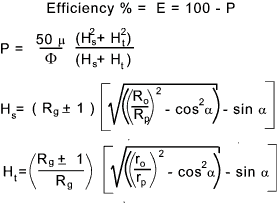Clarification of formula terms.

 Gear Type Φ sign of Rg term External spur cos α (Rg+1) Internal Spur α (Rg-1) Single Helical cos φn / cos 2 β tan φn = tan α.cos β β = helix angle (Rg+1)
Worm Gears

The theoretical efficiency of a worm gear is provided on page worm gears and is shown again below as.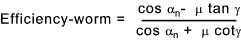Chart of Worm Gear Efficiency

The graph below shows a worm gear efficiencies plotted against the lead angle for different coefficient of friction

Notes:
This chart agrees with the equation for worm gears having a normal pressure angle of 20 degrees...
An approximation for the friction coefficient for worm gears (Bronze -steel) is

μ = 0,04 vs-0,25

vs = sliding velocity (m/s)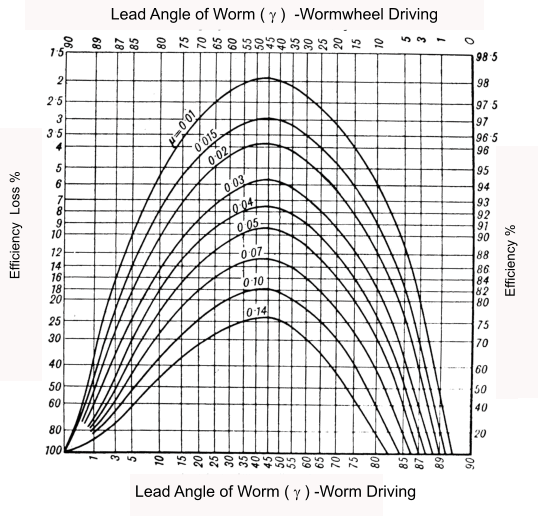Epicyclic Gears

Consider the two meshing gears below which are part of an epicyclic gear train.  This is provided as and example of a typical component in an epicyclic gear.   The two gears and the arms are rotating as shown .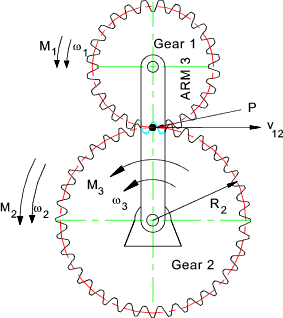Now it the arm was stationary the contact point P would have a instantaneous velocity = -ω2 R2. (velocities to the right are positive ).   Now if the whole system was rotating as a rigid assembly with the velocity of the arm the instantaneous velocity -ω3 R2.  Combining these two motions together, the linear velocity of the tooth engagement (gear 2 -> gear 1)is therefore

v12 = - ( ω2 R2 - ω3 R2)   =    - (ω2 - ω 3)R 2

Now the magnitude of the transmitted tangential force F12 x the tooth engagement velocity v12 is called the potential power and the power loss due to tooth friction is proportional to this power.  Generally for spur gears ( and helical gears) it is sufficient to estimate the power loss as 1% of the potential power. For more accurate estimations the equations above can be used.

P = 0,01 F12v12

The potential power is not the actual power but is the but is the power transmitted by the same gears operating on fixed centres at angular velocities of ( ω2 - ω3 ) for gear 2 and ( ω1 - ω3 ) for gear 2.

The actual pitch line velocity of the gear mesh is -(ω2) and therefore the ration of the potential power to the actual power is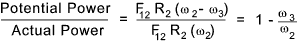Now in cases where the arm is rotating faster than the gear the potential power can be greater than the actual power and the losses proportionally greater.

Example Epicyclic Gear efficiency calculation.

Consider the epicyclic gear chain shown below.   The input speed = 250 RPM (ACW) and the input torque = 2.5 Nm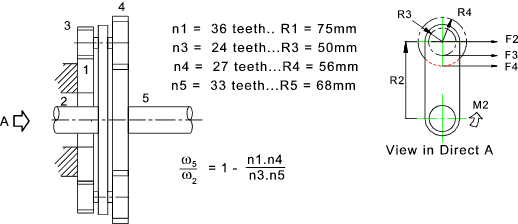ω2 = (250.2.π)/60 = 26,18rads/s.    and    ω1 = 0
The power into the gear = Tω2 = 2,5.26,18 = 65 Watts. Calculating forces
The torque on the arm 2 = M2 = 2.5Nm
The force (F2) at Radius R2 (= R1 + R3) : = -(M2/R2) : F2 = -2.5 /(0,1m+.025m) = -20 N (forces to right are positive)
Now all forces and torques on the link between Arm(2) and gears (3) & (4) are in equilibrium therefore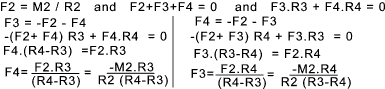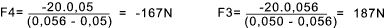For the gear mesh between gear 3 and the fixed sun 2 the velocity of tooth engagement is calculated by the product of the angular velocity of the arm 2 at pitch radius of R2. = ω2R1 = 26,18.0,075 = 1,96m/s

The potential power relates to the gear pair operated in isolation -
in this case for gear 3 to be in equilibrium (With forces and moments)
F2.R3 + F3.R3 = 0 and F2.R3 + F3R3 = 0 therefore F3 = -F2 = 20N
The potential power of the mesh between the sun gear 1 and the planet gear 3
= the gear engagement velocity(1,96m/s) x the tangential force (F3= 20N) = 1,96.20 =3,92W
Allowing 1% loss on a typical spur gear engagement the power loss at this engagement = 0,39W.

For the gear engagement between the gears 4 and 5 the angular velocity of gear five =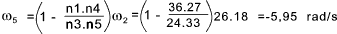The velocity of tooth engagement between gear (4) and gear (5) =
R5 (ω5 - ω2) = 0,068(-5,95- 26,18)=-2.18 m/s

The potential power at this tooth engagement =
the gear engagement velocity (2,18 m/s). x the tangential force (F4 = -167N) = 2,18.167 = 364 W
Assuming 1% power loss then the loss at this tooth engagement = 3,64 W

The power input to the epicyclic gear = 65 W therefore the Gear efficiency = 100(1 - (3,64 + 0,39) /65 ) = 94%

 Links to Gear Efficiency SDP/SI Technical Library...Lots of useful download documents with information of gear efficiency Gear Efficiency comparison .. Short comparison - A second look at GearBox efficiencies .. A short informative download paper on spur gears /motors -

Drive_Index